# Rotational and Vibrational Spectra of Diatomic Molecules - Molecular Spectroscopy, CSIR-NET Notes - Government Jobs

## Government Jobs: Rotational and Vibrational Spectra of Diatomic Molecules - Molecular Spectroscopy, CSIR-NET Notes - Government Jobs

The document Rotational and Vibrational Spectra of Diatomic Molecules - Molecular Spectroscopy, CSIR-NET Notes - Government Jobs is a part of Government Jobs category.
All you need of Government Jobs at this link: Government Jobs

Introduction

Free atoms do not rotate or vibrate. For an oscillatory or a rotational motion of a pendulum, one end has to be tied or fixed to some point.  In molecules such a fixed point is the center of mass.  The atoms in a molecule are held together by chemical bonds.  The rotational and vibrational energies are usually much smaller than the energies required to break chemical bonds. The rotational energies correspond to the microwave region of electromagnetic radiation (3x1010 to 3x1012 Hz; energy range around 10 to100 J/mol) and the vibrational energies are in the infrared region (3x1012 to 3x1014 Hz; energy range around 10kJ/mol) of the electromagnetic radiation.  For rigid rotors (no vibration during rotation) and harmonic oscillators (wherein there are equal displacements of atoms on either side of the center of mass) there are simple formulae characterizing the molecular energy levels.  In real life, molecules rotate and vibrate simultaneously and high speed rotations affect vibrations and vice versa. However, in our introductory view of spectroscopy we will simplify the picture as much as possible.  We will first take up rotational spectroscopy of diatomic molecules.

Rotational Spectra of diatomics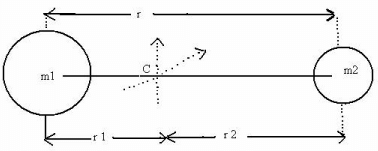Fig.13.1.   A rigid diatomic with masses m1  and m2  joined by a thin rod of length r = r1 + r2 .The centre of mass is at C.

The two independent rotations of this molecule are with respect to the two axes which pass though C and are perpendicular to the “bond length” r.  The rotation with respect to the bond axis is possible only for “classical” objects with large masses.  For quantum objects, a “rotation” with respect to the molecular axis does not correspond to any change in the molecule as the new configuration i s indistinguishable from the old one.

The center of mass is defined by equating the moments on both segments of the molecular axis.

m1 r2= m2 r         (13.1)

The moment of inertia is defined by

I  =  m1 r12 + m2 r22       (13.2)

= m2 r2r1 + m1r1r2

= r1r2  (m1 + m2)      (13.3)

Since  m1 r1  = m2 r2 =m2 (r - r1) ,(m1 + m2) r1 = m2r    Therefore,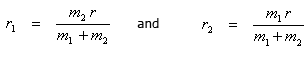(13.4)

Substituting the above equation in (13.3), we get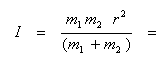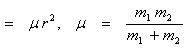(13.5)

Where μ, the reduced mass is given by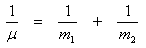(13.6)

The rotation of a diatomic is equivalent to a “rotation” of a mass μ at a distance of r from the origin C. The kinetic energy of this rotational motion is K.E. =  L2/2I   where L is the angular momentum,  Iω where ω is the angular (rotational) velocity in radians/sec.  The operator for L2 is the same as the operator L2 for the angular momentum of hydrogen atom and the solutions of the operator equations L2 Υlm  =  l (l + 1) Ylm, where Ylm are the spherical harmonics which have been studied in lecture 3.

The quantized rotational energy levels for this diatomic are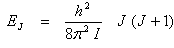(13.7)

The energy differences between two rotational levels is usually expressed in cm-1.  The wave number corresponding to a given ∆E  is given by

ν  =  ∆E /hc,   cm-1    (13.8)

The energy  levels in cm-1 are therefore,

Ej = B J (J +1) where B =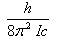(13.9)

The rotational energy levels of a diatomic molecule are shown in Fig. 13.2.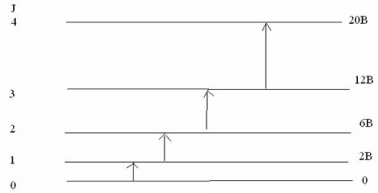Fig. 13.2   Rotational energy levels of a rigid diatomic molecule and the allowed  transitions.

The selection rule for a rotational transition is,

∆ J  =  ± 1      (13.10)

In addition to this requirement, the molecule has to possess a dipole moment.  As a dipolar molecule rotates, the rotating dipole constitutes the transition dipole operator μ. Molecules such as HCl and CO will show rotational spectra while H2, Cl2 and CO2 will not.  The rotational spectrum will appear as follows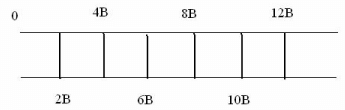Fig. 13.3  Rotational spectrum of a rigid diatomic. Values of B are in cm-1.  Typical values of B in cm-1 are 1.92118 (CO),  10.593 (HCl),  20.956 (HF),  1H2 (60.864),  2H2 (30.442), 1.9987 (N2).

From the value of B obtained from the rotational spectra, moments of inertia of molecules I, can be calculated.  From the value of I, bond length can be deduced.

Example 13.1: Calculate the value of I and r of CO.  B = 1.92118  cm-1 .

Solution:

I = h/(8π2 Bc)  =  6.626 x 10 -34/(8 x 3.14152 x 1.92118 x 3 x 1010)

=  1.45579 x 10-46 kg m

Since the value of B is in cm-1, the velocity of light c is taken in cm/s.  I = μr2.  The atomic mass of C ≡ 12.0000 amu, O ≡ 15.9994 amu.  1 amu = 1.6604 x 10-27 kg.  The reduced mass of CO  can be calculated to be 1.13836 x 10-27 kg.

Therefore r2  =  I/µ = 1.45579 x 10-46/1.13826 x -27 m2

Or  r = 1.131 Ǻ

The rotational levels are degenerate.  Just as there are three p orbitals for l = 1, for J = 1, there are 3 degenerate rotational states.  The degeneracy for a given value of J is 2J + 1.  The Boltzmann factor gets modified due to this degeneracy as follows

NJ/NJ′ =  [(2J + 1)/(2J′ + 1)] e-ΔE/kB    (13.11)

The implication of this is that the rotational population of the J = 1 level is often more than the population of the J = 0 levels since their degeneracies are 3 and 1 r espectively. W hen molecules rotate with great speeds, they cannot be treated as rigid any more. There are distortions due to centrifugal and other forces.  The modification of rotational energies by considering the centrifugal distortion alone is

EJ ( in cm-1)  =  B J(J +1) – DJ2(J+1)2       (13.12)

Where the centrifugal distortion constant D is given by

D = h3/(32π4 I2 r2 kc)  cm-1     (13.13)

The only new term in Eq (13.13) is the force constant k which will be discussed when we study molecular vibrations.

Rotational Spectra of Polyatomics

Linear molecules such as OCS and HC≡CCl  have spectra similar to diatomics.  In diatomics as well as linear triatomics,  IA = IB; IC = 0.   IA, IB and IC are the three moments of inertia of molecules along three independent axes of rotation.  Just as any translation can be decomposed into three independent components along three axes such as x, y and z, any rotation can be decomposed into rotations along three axes A, B, and C.  The way to choose these axes is to have the simplest values of IA, IB and IC. Since triatomics are heavier than the constituent diatomics, their moments of inertia are larger and the values of rotational constants, B, are smaller, in the range of 1 cm-1.  The value of IA or IB determined from the B value gives the total length of the triatomic.  To determine the two bond lengths in the linear triatomic, we need to determine the moment of inertia IA' of an isotope of the triatomic.  From two values of IA and IA' , we can determine the two bond lengths.

The rotational spectra of asymmetric molecules for whom IA ≠ IB ≠ IC can be quite complicated. For symmetric tops, two moments of inertia are  equal ie.,

IA = IB ≠ IC ;     IC ≠  0    (13.14)

In CH3Cl for example, the main symmetry axis is the C – Cl axis.  We need two quantum numbers to describe the rotational motion with respect to IA and IC respectively.  Let J represent the total angular momentum of the molecule and K the angular momentum with respect to the C – Cl axis of the symmetric top.  J takes on integer values and K can not be greater than J (recall that ml≤ l |  for orbital angular momentum).  The (2J + 1) “degeneracy” is expressed through the 2J + 1 values that K can take.

K  =  J,  J – 1, …..  0,         -  (J – 1),  - J      (13.15)

The rotational energies of a symmetric top are given by

Ej k / hc = BJ(J +1) + (A-B) K2, cm-1        (13.16)

The moments of intertia are related to B and A as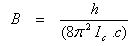and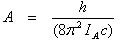(13.17)

As the energy depends on K2, energies for states with + K and – K are doubly degenerate. Thus there will be J + 1 levels and (2J + 1) states for each values of J.

The selection rules for the symmetric top are,

ΔJ  =  ± 1 and ΔK = 0     (13.18)

It can be easily shown that

EJ +1, K – E J,,K)/hc  = 2 BJ (J +1)    (13.19)

This implies that the spectrum is independent of the value of K.  The physical meaning is as follows.  K refers to the rotation about the symmetry axis such as the C - Cl axis.  A rotation about this axis does not change the dipole moment.  We mentioned in the section on the rotational spectra of diatomics that the molecular dipole moment has to change during the rotational motion (transition dipole moment operator of Eq 12.5) to induce the transition. Rotation along the axis A and B changes the dipole moment and thus induces the transition.

By using rotational or microwave spectroscopy, very accurate values of bond lengths can be obtained. For example, in HCN, the C-H length is 0.106317 ± 0.000005 nm and the CN bond length is 0.115535 ± 0.000006 nm.  The principle of the microwave oven involves heating the molecules of water through high speed rotations induced by microwaves. The glass container containing water however remains cold since it does not contain rotating dipoles.

Vibrations and Rotations of a diatomic

You have noticed in your earlier studies that simple pendulums or stretched strings exhibit simple harmonic motion about their equilibrium positions.  Molecules also exhibit oscillatory motions.  A diatomic oscillates about its equilibrium geometry.  The quantized vibration energies  Eυ of a harmonic oscillator are

Eυ = (u + ½) hν    (13.20)

v = 0,1,2,…………

The vibrational frequency  ν is related to the force constant k through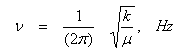(13.21)

The vibrational motion occurs under the action of a binding potential energy. The potential energy (PE) curve for a harmonic oscillator is given in Fig. 13.4.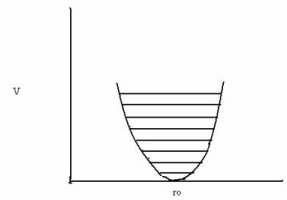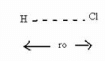Figure 13.4. The potential energy of a harmonic oscillator V = k(r-ro)2.  The force constants k in N/m for a few moleculues are, CO (1902), HF (966), HCl (516), HI (314).

On either sides of the equilibrium bond length r0, the PE rises as a symmetric quadratic function (a parabola).  The vibrational wavefunctions can be obtained by solving the Schrodinger equation.  The Hamiltonian operator (for energy) now consists of a kinetic energy term and a potential energy term V as shown in Fig. 13.4 and the solutions for energy, Ev have already been given in Eq.(13.20).  The selection rules for the harmonic oscillator are:

Δv = ± 1          (13.22)

We will see  several equally spaced lines (spacing hν) corresponding to the transitions 0→1, 1→2,  2→3 and so on.  The first transition will be the most intense as the state with v = 0 is the most populated. In actual diatomics, the potential is anharmonic.  A good description of an anharmonic oscillator is given by the Morse function.

P.E.  =  Deq [1 – exp {a(ro -r }] 2    (13.23)

In Eq. (13.22), Deq is the depth of the PE curve and r0 is the bond length.  A plot of the Morse curve and the energy levels for the Morse potential are given in Fig. 13.5.  The formula for the energy levels of this anharmonic oscillator is

Ev/ hc = ev = (v + ½) ν -  (v + ½)2 ν x e,  cm-1      (13.24)

Here xe, is called the anharmonicity constant whose value is near 0.01.  It can be easily deduced from the above formula that the vibrational energy levels for large υ start bunching together.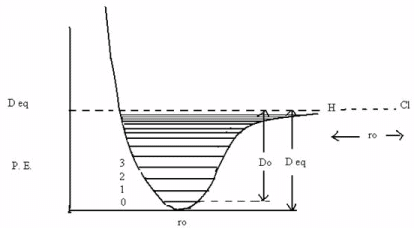Fig. 13. 5.   The Morse potential and the energy levels therein.  Note the difference between the dissociation energy Do and the depth Deq.  All molecules have a minimum of the zero point energy of hν/2 corresponding to the ν = 0 state.  This is a consequence of the uncertainty principle!

Often, one observes a combined vibrational rotational spectrum.  A combined set of vibrational and rotational energy levels of a diatomic is given by

Etotal =  BJ (J + 1) + ( v + ½ ) ν -  xe (v + ½)2  ν,   cm-1   (13.25)

The energy level diagram and the spectrum corresponding to the diagram are shown in Fig.  (13.6).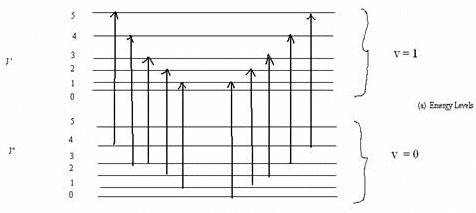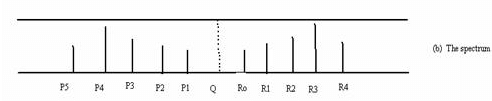Fig. 13.6.  The vibrational rotational spectrum.

The selection rules are  ∆v = ± 1, ± 2,…

∆ J  =  ± 1.   ∆ J = 1 corresponds to the R branch on the right at higher frequencies and

∆ J = J” – J’  = -1  corresponds to the P branch on the left.  The dashed line Q for which

∆ J  = 0, is not seen.  The difference between R0 and P1 is 4B and the difference between adjacent R

Vibrational spectra of Polyatomics

An atom moving in three dimensions has three degrees of freedom corresponding to the freedom in movement in, say, the x, y and z directions.  A collection of N unabound atoms will have  3N degrees of freedom.  If the N atoms are bound through the formation of a molecule, the 3N degrees of freedom get redistributed into translational rotational and vibrational modes.  Since the molecule can be translated as a unit, there are three translational modes (degrees of freedom).  Similarly there are three rotational modes with respect to three independent axis of rotation.  The remaining, 3N-6 are the vibrational modes.  For a linear molecule, since there are only two rotational modes with respect to the two axes perpendicular to the molecular axis, there are 3N-5 vibrational modes.

If the potentials energy functions for all the motions can be assumed to be harmonic, then the 3N-6 modes can be categorized into 3N-6 normal modes. Consider the example of water.  There are three atoms and 3N-6 = 3 normal modes.  In terms of the potential energy functions for vibrations, there are three functions: one each corresponding to each O-H bond and one corresponding to the H-O-H bending.  In terms of the individual bond vibrations, the vibrational motion can appear quite complex. The total potential energy P.E. may be written as:

P.E. = ½ k (r1 -r10)2 + ½ k (r2 – r20)2 + ½k ′(θ-θ0 )2      (13.26)

Here, r10 and r20 are the equilibrium bond lengths of the two O-H bonds and θ0 is the equilibrium bond angle.  A normal mode of vibration is defined as a vibration in which all atoms oscillate with the same frequency and pass through their equilibrium positions at the same time.  The center of mass is unchanged during a normal mode.  The three normal modes of vibration of water are shown in the following figure.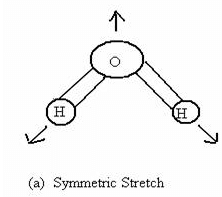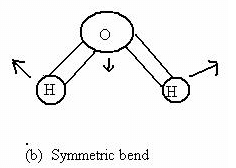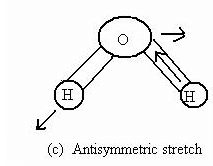Figure 13.7  Normal modes of vibrations of water

The three normal modes of vibrations of water (Fig 13.7) are the symmetric stretch (ν1 = 3651.7 cm-1), the antisymmetric stretch (ν2 = 3755.8 cm-1)  and the symmetric bend  (ν3 = 1595.0 cm-1).  Bending requires less energy and thus, its frequency is lower.  The asymmetric stretch requires greater reorganization than the symmetric stretch and hence a larger frequency.  Molecular CO2 is a linear triatomic and has  3N - 5 = 4 normal modes of vibration.  The symmetric stretch (ν1 = 1330 cm-1) asymmetric stretch (ν2 = 2349.3 cm-1) and bending (ν3 = 66.3 cm-1) are shown in Fig 13.8.  The bending mode is doubly degenerate, owing to the two independent bending modes in two perpendicular planes containing the molecular axis.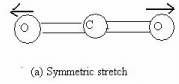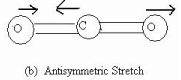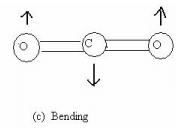Figure 13.8  Normal modes of vibrations of CO2

Different molecules can be easily identified by their normal mode frequencies.  In addition to these modes, overtones (2ν1, 3ν2, etc.),  combination bonds (ν1 + ν2, 2ν1 + ν2, ν123 …),  and difference bands (ν1 - ν2,  ν12 - ν3) can be observed.  Since a large number of rotational and vibrational levels are closely spaced they provide a rich base for setting up lasers when the upper levels are populated.  As in the case of diatomics, rotational lines are richly dispersed in vibrational spectra of polyatomics.  The concept of normal modes can be extended to solids and liquids too.  Since in a solid, there are a very large number of atoms (of the order of Avogadro number), there are 3N-6 normal modes.  These are characterized as phonons, which correspond to collective motions of atoms in a solid.

Analysis by IR Spectroscopy

IR spectroscopy has grown into an extremely versatile analytical tool.  Most organic and inorganic groups (such as CH3, -C=C, M-C≡0) have characteristic frequencies and these frequencies provides finger prints, using which the groups in newly synthesized molecules can be identified.  Although we can not “see” molecules, through various spectral methods, we can identify atoms, groups, bond lengths, relative locations (cis/trans, endo/exo) and so on.  The IR frequencies of a few common groups are given in Table 13.2.

Table 13.2  Characteristic frequencies (in cm-1) of some molecular groups.

 Group Approximate frequency Group Approximate frequency C-I 550 -C = C - 2200 C-Cl 725 -C = N 2250 C=S 1100 S - H 2580 C-O- 1000 - 1200 -CH2 2930 (asym stretch) 2860 (sym stretch) C-N 1000 - 1200 1470 (deformation) C-C 1000 - 1200 = CH2 3030 C=N- 1600 Aromatic C-H 3060 C = C 1650 ≡C – H 3300 C = O 1600 – 1750 -N-H2 3400 O-H 3600 H-bonds 3200 – 3570

In special conformations, the group frequencies can deviate from the values in the table significantly.

The document Rotational and Vibrational Spectra of Diatomic Molecules - Molecular Spectroscopy, CSIR-NET Notes - Government Jobs is a part of Government Jobs category.
All you need of Government Jobs at this link: Government JobsUse Code STAYHOME200 and get INR 200 additional OFF

Track your progress, build streaks, highlight & save important lessons and more!

,

,

,

,

,

,

,

,

,

,

,

,

,

,

,

,

,

,

,

,

,

,

,

,

;